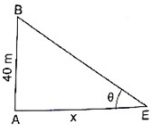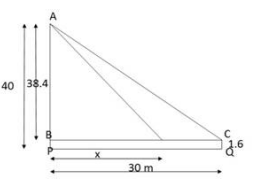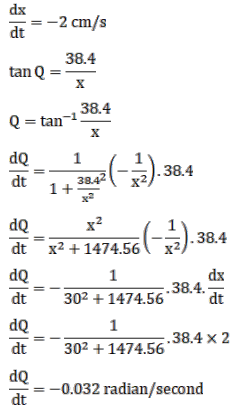# A man is moving away from

Question:

A man is moving away from a $40-\mathrm{m}$ high tower at a speed of $2 \mathrm{~m} / \mathrm{s}$. Find the rate is which the angle of elevation of the top of the tower is changing when he is at a distance of 30 metres from the foot of the tower. Assume that the eye level of the man is $1.6 \mathrm{~m}$ from the ground.Solution: